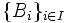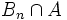# Second-countability is hereditary

This article gives the statement, and possibly proof, of a topological space property (i.e., second-countable space) satisfying a topological space metaproperty (i.e., subspace-hereditary property of topological spaces)
View all topological space metaproperty satisfactions | View all topological space metaproperty dissatisfactions
Get more facts about second-countable space |Get facts that use property satisfaction of second-countable space | Get facts that use property satisfaction of second-countable space|Get more facts about subspace-hereditary property of topological spaces

## Statement

### Property-theoretic statement

The property of topological spaces of being a second-countable space satisfies the metaproperty of topological spaces of being hereditary.

### Verbal statement

Any subspace of a second-countable space is second-countable under the subspace topology.

## Definitions used

### Second-countable space

Further information: Second-countable space

A topological space is termed second-countable if it admits a countable basis.

### Subspace topology

Further information: Subspace topology

Given a topological space$X$ and a subspace$A$, with a basis$\{ B_i \}_{i \in I}$ for$X$, the subspace topology on$A$ is defined as a topology with basis$B_i \cap A$.

## Proof

Given: A second-countable space$X$ with countable basis$B_n, n \in \mathbb{N}$. A subspace$A$ of$X$

To prove:$A$ has a countable basis.

Proof: By the definition of subspace topology, the sets$B_n \cap A$ form a basis for the subspace topology on$A$. This is a countable basis for$A$.